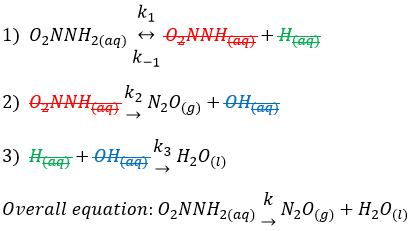# Problem: The decomposition of nitramide, O2NNH2, in water has the following chemical equation and rate law:O2NNH2(aq)→ N2O(g) + H2O(l)rate= [O2NNH2]/[H]A proposed mechanism for this reaction is:k1(1) O2NNH2(aq) ↔ O2NNH(aq) + H(aq)  (fast equilibrium) k-1    k2(2) O2NNH(aq) → N2O(g) + OH(aq)  (slow)    k3(3) H(aq) + OH → H2O(l)  (fast)What is the relationship between the observed value of k and the rate constants for the individual steps of the mechanism?k = __________Which symbols go into the numerator or denominator: k-1, k3, k2, k1.

###### FREE Expert Solution
94% (467 ratings)
###### FREE Expert Solution

The problem is about the decomposition of nitramide (O2NNH2) in water and we’re asked to determine two things:

• Determine the relationship between the observed value of k and the rate constants for the individual steps of the mechanism.
• To choose the correct symbols for k.

First, remember that the Elementary steps of the mechanism must result in the overall reaction:

So we add the 3 reaction steps and cancel terms in both reactants and products.94% (467 ratings)###### Problem Details

The decomposition of nitramide, O2NNH2, in water has the following chemical equation and rate law:

O2NNH2(aq) N2O(g) + H2O(l)

rate= [O2NNH2]/[H]

A proposed mechanism for this reaction is:

k1

(1) O2NNH2(aq)  O2NNH(aq) + H(aq)  (fast equilibrium)

k-1

k2

(2) O2NNH(aq) → N2O(g) + OH(aq)  (slow)

k3

(3) H(aq) + OH  H2O(l)  (fast)

What is the relationship between the observed value of k and the rate constants for the individual steps of the mechanism?

k = __________

Which symbols go into the numerator or denominator: k-1, k3, k2, k1.